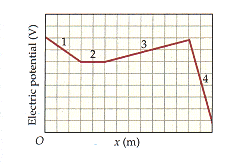# Electric Potential, Electric Potential Energy, Electric Field

MozAngeles

## Homework Statement

As a +6.2-µC charge moves along the x-axis from x = 0 to x = 1.4 m, the electric potential it experiences is shown in the figure, below. (The horizontal axis is marked in increments of 0.1 m and the vertical axis is marked in increments of 5 V.) Find the approximate location(s) of the charge when its electric potential energy U equals the the following values. (If there are more than one answer, enter your answers from smallest to largest. If there is only one answer, enter your answer in the first answer box and enter "none" in the following boxes.)

Here is the graph: http://www.webassign.net/walker/20-22alt… [Broken]

(a) U = 0.000124 J
x1 = m
x2 = m
x3 = m

(b) U = 0.000217 J
x1 = m
x2 = m
x3 = m

## The Attempt at a Solution

E=-$\Delta$V/$\Delta$x and F=Eq and W=Eqd and W= -$\Delta$U. So for the a). X1 i found the slope for region one which I found to be 50, then I used the Force equation and got 3.81e8 N then plugging those values into my work equation, solving for d I got 4e-13. But this is wrong any help in the right direction would be nice.. Thanks in advance.

Last edited by a moderator: